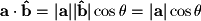A component of a vector is a scalar value which represents the magnitude of a vector along a certain direction. So far when we have referred to a vector's magnitude, we have been finding the magnitude along the vector's direction. If you have had previous experience with vectors, you may be familiar with finding the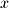- and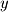-components as shown in Figure 2-8 which represent the magnitude of the vector in the direction of theandaxes respectively.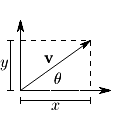Figure 2-8.andComponents of a Vector
These values are easy to find using basic trigonometry since the axes form a right triangle: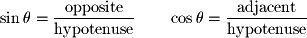Ifis the angle between the x-axis and the vector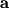and we know that the length of the hypotenuse is the magnitude of the vector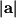, then the x-component is the length of the adjacent side and the and the y-component is the length of the opposite side: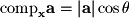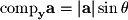If you do not already know these relationships, you should memorize them now (keep in mind they are easy to remember if you know the definition of sine and cosine).

In linear algebra we sometimes need to find the component of a vector in a direction other than the x and y axes. In Figure 2-9, we have two vectorsand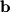and we want to know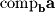(the component ofin the direction of). Again we can form a right triangle with the two vectors and we find the following whereis the angle between the two vectors: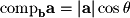Note that this formula is the same as the formula for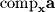because in the previous examplewas the angle betweenand the x axis and in this caseis the angle betweenand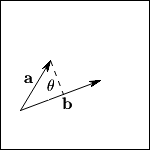Figure 2-9.Component of VectorIn linear algebra it is more common to define the component formula using the dot product. In order to do this we have to normalizeto the unit vector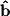so that the magnitude in the dot product equals 1: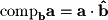Substituting the geometric interpretation of the dot product into this formula gives the following which is what we obtained previously: